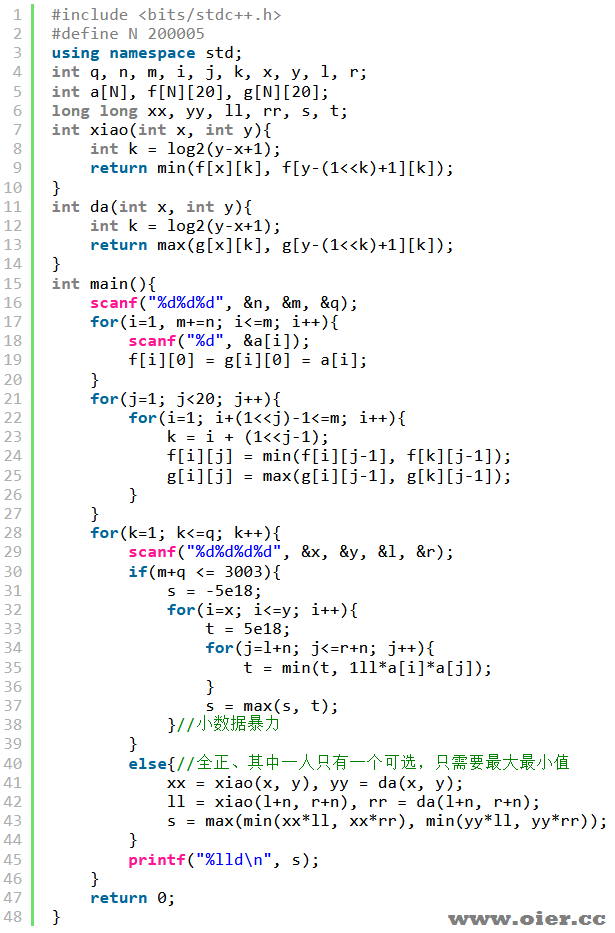474+

## 输入输出样例

### 输入样例 #1

3 2 2
0 1 -2
-3 4
1 3 1 2
2 3 2 2


### 输出样例 #1

0
4


### 输入样例 #2

6 4 5
3 -1 -2 1 2 0
1 2 -1 -3
1 6 1 4
1 5 1 4
1 4 1 2
2 6 3 4
2 5 2 3


### 输出样例 #2

0
-2
3
2
-1


## 说明

**【样例解释 \#1】**

$$\begin{bmatrix} 0 & 0 \\ -3 & 4 \\ 6 & -8 \end{bmatrix}$$

**【样例 \#3】**

**【样例 \#4】**

**【数据范围】**

| 测试点编号 | $n, m, q \le$ | 特殊条件 |
|:-:|:-:|:-:|
| $1$ | $200$ | 1, 2 |
| $2$ | $200$ | 1 |
| $3$ | $200$ | 2 |
| $4 \sim 5$ | $200$ | 无 |
| $6$ | $1000$ | 1, 2 |
| $7 \sim 8$ | $1000$ | 1 |
| $9 \sim 10$ | $1000$ | 2 |
| $11 \sim 12$ | $1000$ | 无 |
| $13$ | ${10}^5$ | 1, 2 |
| $14 \sim 15$ | ${10}^5$ | 1 |
| $16 \sim 17$ | ${10}^5$ | 2 |
| $18 \sim 20$ | ${10}^5$ | 无 |

## 程序实现ST表85分代码：写太多ST表会很累，剩下15分不要了，或者写线段树吧！